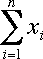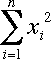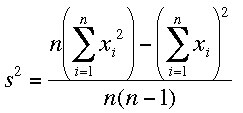SEARCH HOMEMath Central Quandaries & QueriesIn relation my question asked earlier, i would first like to thank you for the prompt reply. But it has not answered my query yet. Let me frame the problem again. I have a mean value for N data items. (note that i know N but i donot know the distinct N data item values) Now the (N+1)th data item comes in and i calculate the new mean. (incremental method for that is simple). How do i calculate the new standard deviation. The formula you gave me requires me to read the data items once and at the end of the pass i will have have the standard deviation. But i need the STDDEV values at intermediate stages as well. Is it possible ? MurtazaMurtaza,

You know the mean and variance s2 (square of the standard deviation) and the number of items in the data set n. Let

Sx =and SSx =Sx is n times the mean so you can find the value of Sx as n times the mean. Once you have this value then using the expression for the variance in my earlier responseyou can find

SSx = (n-1) s2 + 1/n Sx2

Now suppose you are given a new x value, xn+1 As you remarked you can find the sum of the x values as

newSx = Sx + xn+1

and hence the new mean is

1/(n+1)newSx

But you can also find the new SSx by

newSSx = SSx + xn+12

and

new Variance = 1/[(n+1)n][(n+1)newSSx - (newSx)2]

I hope this helps,
PennyMath Central is supported by the University of Regina and The Pacific Institute for the Mathematical Sciences.